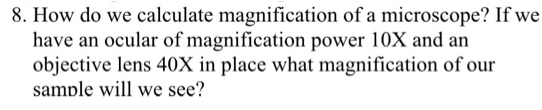# Problem: How do we calculate magnification of a microscope? If we have an ocular of magnification power 10X and an objective lens 40X in place what magnification of our sample will we see?

###### FREE Expert Solution

The calculation of the total magnification follows a simle multiplication of each component's individual magnification (i.e. the magnification of the ocular and the objective lens in use.###### Problem Details

How do we calculate magnification of a microscope? If we have an ocular of magnification power 10X and an objective lens 40X in place what magnification of our sample will we see?